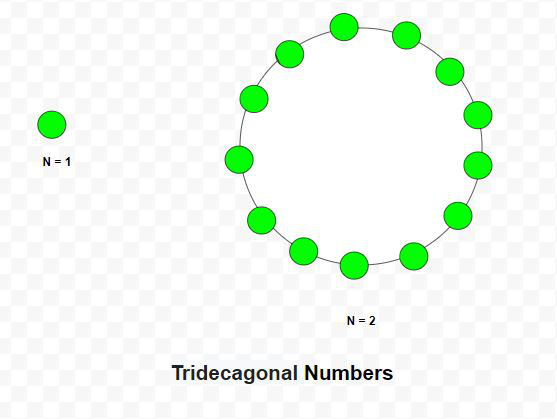Related Articles

# Tridecagonal Number

• Last Updated : 17 Mar, 2021

Given a number N, the task is to find the Nth Tridecagonal number

A tridecagonal number is a figurate number that extends the concept of triangular and square numbers to the tridecagon(a thirteen-sided polygon). The Nth tridecagonal number counts the number of dots in a pattern of N nested tridecagons, all sharing a common corner, where the ith tridecagon in the pattern has sides made of ‘i’ dots spaced one unit apart from each other. The first few tridecagonal numbers are 1, 13, 36, 70, 115, 171 …

Examples:

Input: N = 2
Output: 13
Explanation:
The second tridecagonal number is 13.
Input: N = 6
Output: 171Approach: The Nth tridecagonal number is given by the formula:

``

Below is the implementation of the above approach:

## C++

 `// C++ program to find N-th``// Tridecagonal number` `#include ``using` `namespace` `std;` `// Function to find N-th``// Tridecagonal number``int` `Tridecagonal_num(``int` `n)``{``    ``// Formula to calculate nth``    ``// Tridecagonal number``    ``return` `(11 * n * n - 9 * n) / 2;``}` `// Driver Code``int` `main()``{``    ``int` `n = 3;``    ``cout << Tridecagonal_num(n) << endl;``    ` `    ``n = 10;` `    ``cout << Tridecagonal_num(n) << endl;` `    ``return` `0;``}`

## Java

 `// Java program to find N-th``// tridecagonal number``class` `GFG{` `// Function to find N-th``// tridecagonal number``static` `int` `Tridecagonal_num(``int` `n)``{``    ` `    ``// Formula to calculate nth``    ``// tridecagonal number``    ``return` `(``11` `* n * n - ``9` `* n) / ``2``;``}` `// Driver Code``public` `static` `void` `main(String[] args)``{``    ``int` `n = ``3``;``    ``System.out.print(Tridecagonal_num(n) + ``"\n"``);``    ` `    ``n = ``10``;``    ``System.out.print(Tridecagonal_num(n) + ``"\n"``);``}``}` `// This code is contributed by Princi Singh`

## Python3

 `# Python3 program to find N-th``# tridecagonal number` `# Function to find N-th``# tridecagonal number``def` `Tridecagonal_num(n):``    ` `    ``# Formula to calculate nth``    ``# tridecagonal number``    ``return` `(``11` `*` `n ``*` `n ``-` `9` `*` `n) ``/` `2` `# Driver Code``n ``=` `3``print``(``int``(Tridecagonal_num(n)))` `n ``=` `10``print``(``int``(Tridecagonal_num(n)))` `# This code is contributed by divyeshrabadiya07`

## C#

 `// C# program to find N-th``// tridecagonal number``using` `System;` `class` `GFG{` `// Function to find N-th``// tridecagonal number``static` `int` `Tridecagonal_num(``int` `n)``{``    ` `    ``// Formula to calculate nth``    ``// tridecagonal number``    ``return` `(11 * n * n - 9 * n) / 2;``}` `// Driver Code``public` `static` `void` `Main(String[] args)``{``    ``int` `n = 3;``    ``Console.Write(Tridecagonal_num(n) + ``"\n"``);``    ` `    ``n = 10;``    ``Console.Write(Tridecagonal_num(n) + ``"\n"``);``}``}` `// This code is contributed by Rajput-Ji`

## Javascript

 ``
Output:
```36
505```

Attention reader! Don’t stop learning now. Get hold of all the important DSA concepts with the DSA Self Paced Course at a student-friendly price and become industry ready.  To complete your preparation from learning a language to DS Algo and many more,  please refer Complete Interview Preparation Course.

In case you wish to attend live classes with experts, please refer DSA Live Classes for Working Professionals and Competitive Programming Live for Students.

My Personal Notes arrow_drop_up